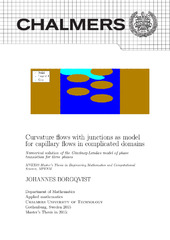## Curvature flows with junctions as model for capillary flows in complicated domains

 dc.contributor.author Borgqvist, Johannes dc.contributor.department Chalmers tekniska högskola / Institutionen för matematiska vetenskaper sv dc.contributor.department Chalmers University of Technology / Department of Mathematical Sciences en dc.date.accessioned 2019-07-03T13:40:02Z dc.date.available 2019-07-03T13:40:02Z dc.date.issued 2015 dc.description.abstract The focus of the thesis is to model capillary ow for a three phase system in complex domains with a model involving curvature ow in combination with triple junctions. The first part of the report involves the implementation of a proposed solution algorithm by Ruuth[16; 17]. The solution algorithm involves two steps, namely a diffusion step that models the curvature ow, and a sharpening step that corresponds to the evolution of the three phase system at the triple junctions, that is, the points where the three phases meet. The diffusion step involves solving the heat equation for a short period of time , and a finite difference approach is implemented in order to solve the heat equation. The sharpening step is conducted by implementing a so called projection triangle algorithm, and this step is also implemented using a finite difference approach. The accuracy of the implementation of the diffusion step is calculated by modelling a two phase system known as "the shrinking sphere" and the projection triangle algorithm is implemented for two different domains. The results of the projection triangle algorithm are compared to the results generated by Ruuth. The second part of the thesis justifies the validity of the projection triangle algorithm in modelling capillary ow. A physical condition concerning the conservation of the con- tact angle is imposed, and a convolution thresholding calculation is presented using the suggested condition. A numerical validation of the convolution thresholding scheme is presented and compared to the results of the projection triangle algorithm. The convo- lution thresholding scheme validates the result from the projection triangle algorithm. The third part of the report includes the numerical simulations of the implemented algo- rithm. The capillary ow for two different liquids are simulated, and the results indicate that a small contact angle corresponds to a faster capillary ow. Furthermore, the capillary ow for the faster of the two liquids is simulated in two different domain, one in R2 and one in R3. The conclusion is that the algorithm proposed by Ruuth can be applied in order to model capillary ow for a three phase system. dc.identifier.uri https://hdl.handle.net/20.500.12380/218493 dc.language.iso eng dc.setspec.uppsok PhysicsChemistryMaths dc.subject Matematik dc.subject Grundläggande vetenskaper dc.subject Mathematics dc.subject Basic Sciences dc.title Curvature flows with junctions as model for capillary flows in complicated domains dc.type.degree Examensarbete för masterexamen sv dc.type.degree Master Thesis en dc.type.uppsok H local.programme Engineering mathematics and computational science (MPENM), MSc